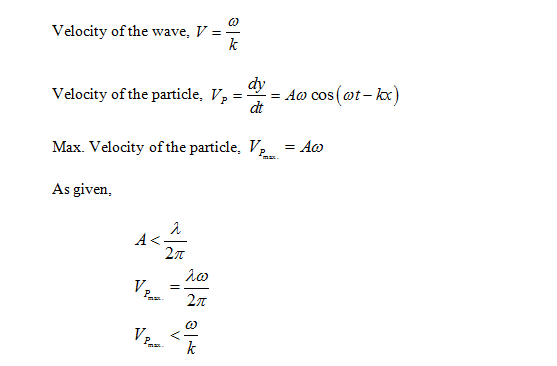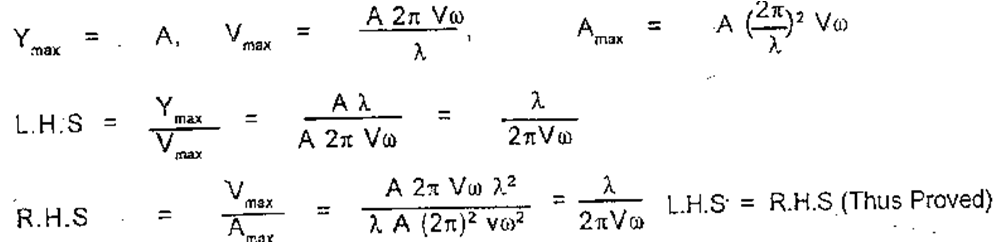# Concepts of Physics Ch-15 Wave Motion and Wave On a String (SA)

1. You are walking along seashore and a mild wind is blowing. Is the motion of air a wave motion?

Solution: No, in wave motion there is no actual transfer of matter but transfer of energy between the points where as when wind blows air particles moves with it.

1. The radio and TV programmes, telecast at the studio, reach our antenna by wave motion. Is it a mechanical wave or non-mechanical?

Solution:  It is non- mechanical wave because this type of wave does not require a material medium to travel.

1. A wave is represented by an equation y= c1 sin (c2x+c3t) . In which direction is the wave going? Assume that c1, c2 and c3 are all positive.

Solution: Equation of the wave is, y= c1 sin (c2x+c3t) when the variable of the equation is (c2x+c3t), and then the wave must be moving in the negative x-axis with time t.

1. Show that the particle speed can never be equal to the wave speed in a sine wave if the amplitude is less than wavelength divided by 2π .

Solution: Equation of the wave is given by, y = A sin (wt-kx); where A is the amplitude, is the angular frequency and k is the wave number.1. Two wave pulses identical in shape but inverted with respect to each other are produced at the two ends of a stretched string. At an instant when the pulses reach the middle, the string becomes completely straight. What happens to the energy of the two pulses?

Solution: When two waves pulses identical in shape but inverted with respect to each other meet at any instant, they form a destructive interference. The complete energy of the system at that instant is stored in the form of potential energy within it. After passing each other, both the pulses regain their original shape.

1. Show that for a wave travelling on a stringwhere the symbols have usual meanings. Can we use componendo and dividendo taught in algebra to writeSolution:(b) We can’t use C and D method since the displacement and velocity can’t be added since they are dimensionally different.

1. What is the smallest positive phase constant which is equivalent to 7.5π?

Solution: The smallest positive phase constant which is equivalent to 7.5π is π/2.

1. A string clamped  at both  ends  vibrates  in  its fundamental mode. Is there any position (except the ends) on the string which can be touched without disturbing the motion ? What if the string vibrates in its first overtone ?

Solution: Yes, at the centre. The centre position is a node. If the string vibrates in its first overtone, then there will be two positions, i.e., two nodes, one at x=0 and the other at x=L.